# Using ggplotly

Making interactive plots

tutorial
Published

July 31, 2022

The function ggplotly is a way to make graphs interactive. It is in the function `ggplot()`. We are going to be making a scatterplot. Let’s go!

## Libraries

I’m loading in my libraries here. I will be using the package tidyverse, which includes ggplot, the package gapminder, that’s the data we’re using, and MetBrewer, one of the solutions for changing the colors.

Code
``````library(tidyverse)
library(gapminder)
library(MetBrewer)
library(plotly)``````

Great! We know what data we’re using, so we can start plotting!

## Plotting

We can start making the plot now. We start it off with `ggplot`, set data equal to gapminder, and we add `lifeExp` to the y axis and `gdpPercap` to the x axis inside of `mapping = aes()`.

Code
``````ggplot(data = gapminder,
mapping = aes(x = gdpPercap,
y = lifeExp))``````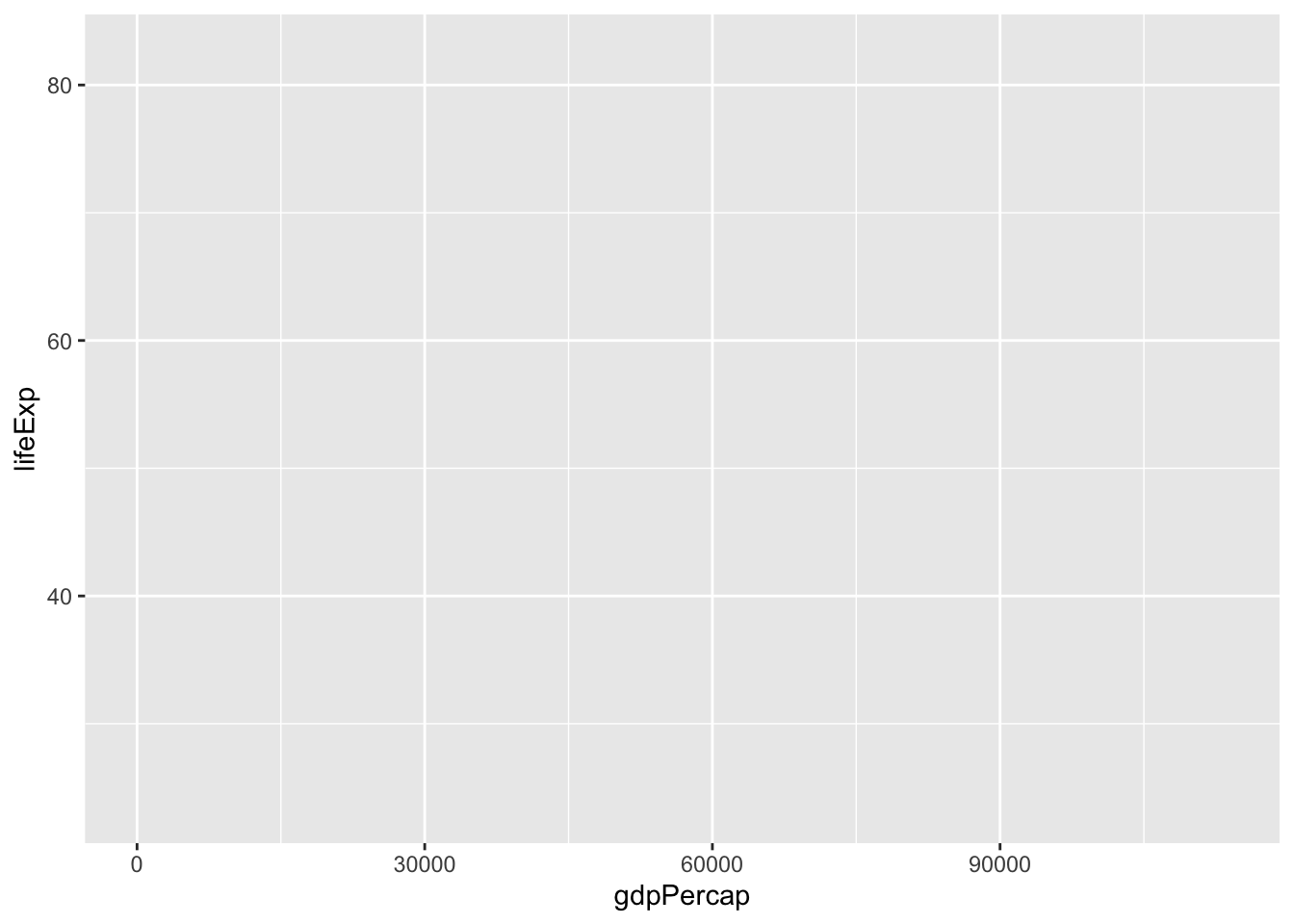Now, we can add the `geom`.

We are going to be using `geom_point()` to make a scatterplot. Add another layer to your graph with ‘+’.

Code
``````ggplot(data = gapminder,
mapping = aes(x = gdpPercap,
y = lifeExp))+
geom_point(alpha = 0.3)``````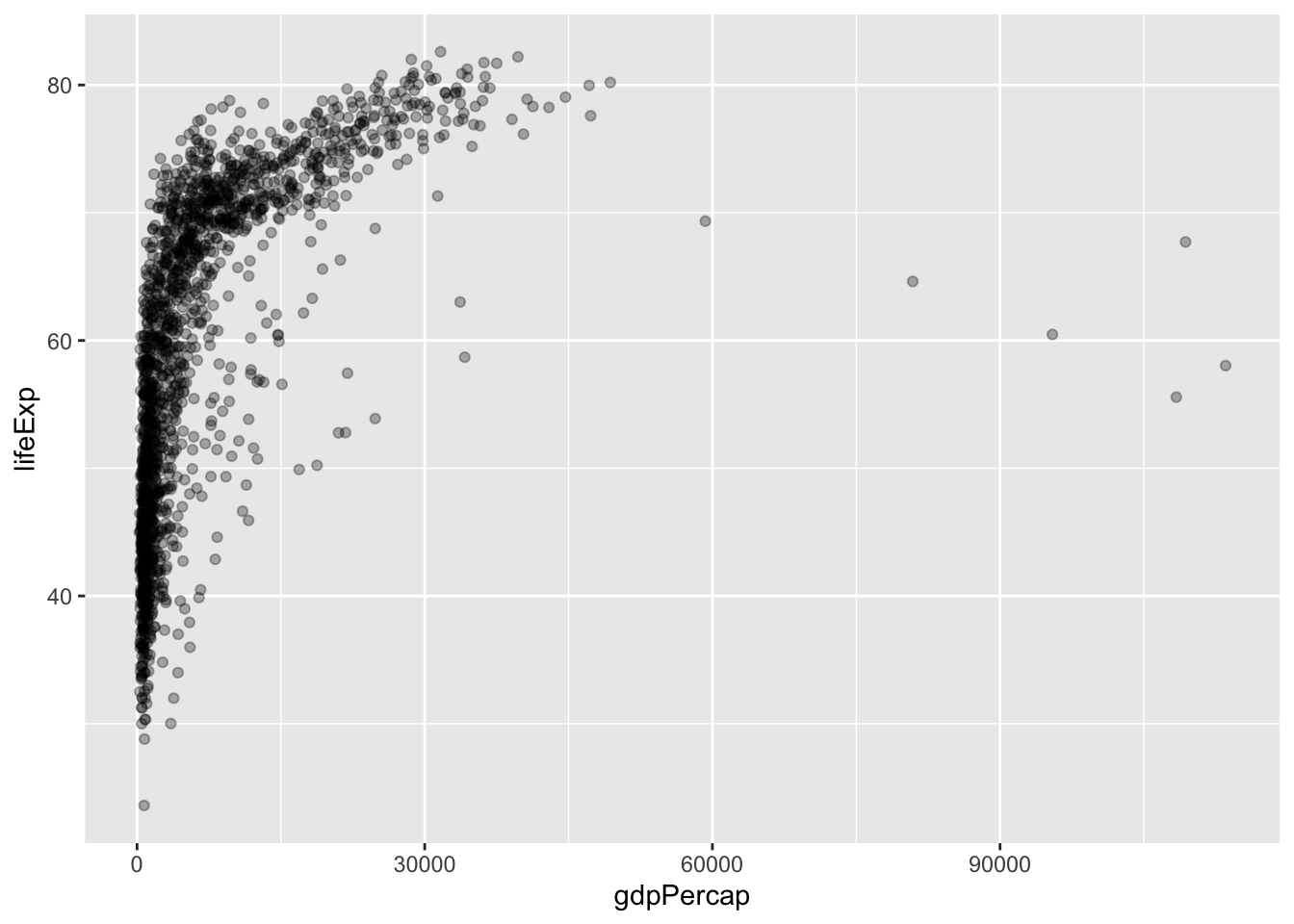That’s just a bunch of black dots. How about we change the colors.

## Changing the Colors

There are many ways to change the colors, I cover 3 ways in my tutorial “How to make a Histogram”. I will just be covering MetBrewer.

Let’s set color equal to continent within `aes()`.

Code
``````ggplot(data = gapminder,
mapping = aes(x = gdpPercap,
y = lifeExp,
color = continent)) +
geom_point(alpha = 0.3)``````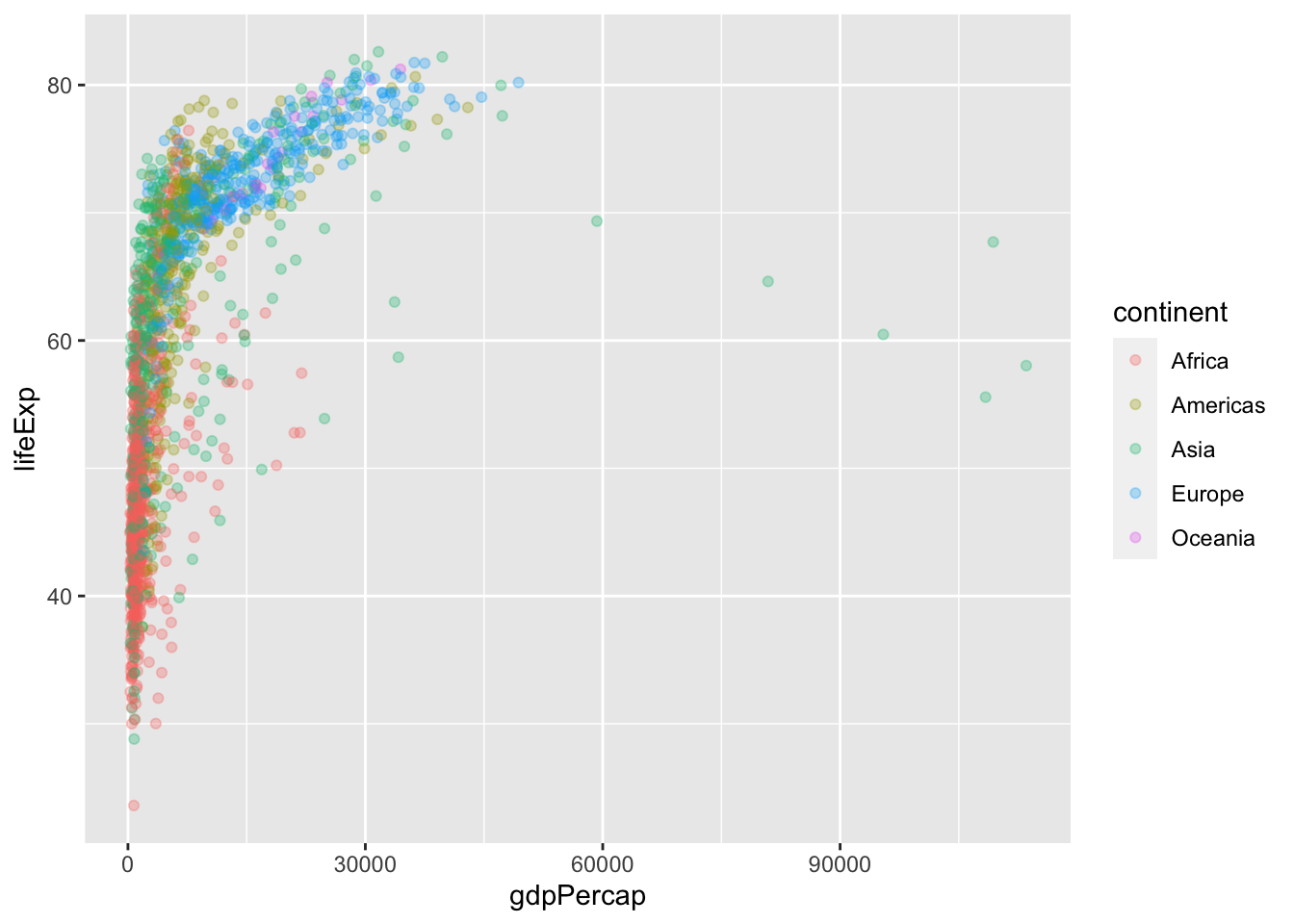Now, we can see clearly what continents are healthier and wealthier. What if we changed the colors to a nicer palette?

### Changing the colors (MetBrewer)

MetBrewer is a package that has beautiful color palettes that are made from art in the MET. We will use one that has 5 distinct colors to use for our plot. I chose “Lakota” and selected 5 colors from it, using a new layer, with `scale_color_manual(values = met.brewer())`. This allows you to make your own list of colors you want for your graph. I also changed the size population, just to make things more interesting. I also got rid of the alpha, but we will fix that soon

Code
``````ggplot(data = gapminder,
mapping = aes(x = gdpPercap,
y = lifeExp,
size = pop,
color = continent)) +
geom_point(alpha = 0.3) +
scale_color_manual(values = met.brewer("Lakota", 5))``````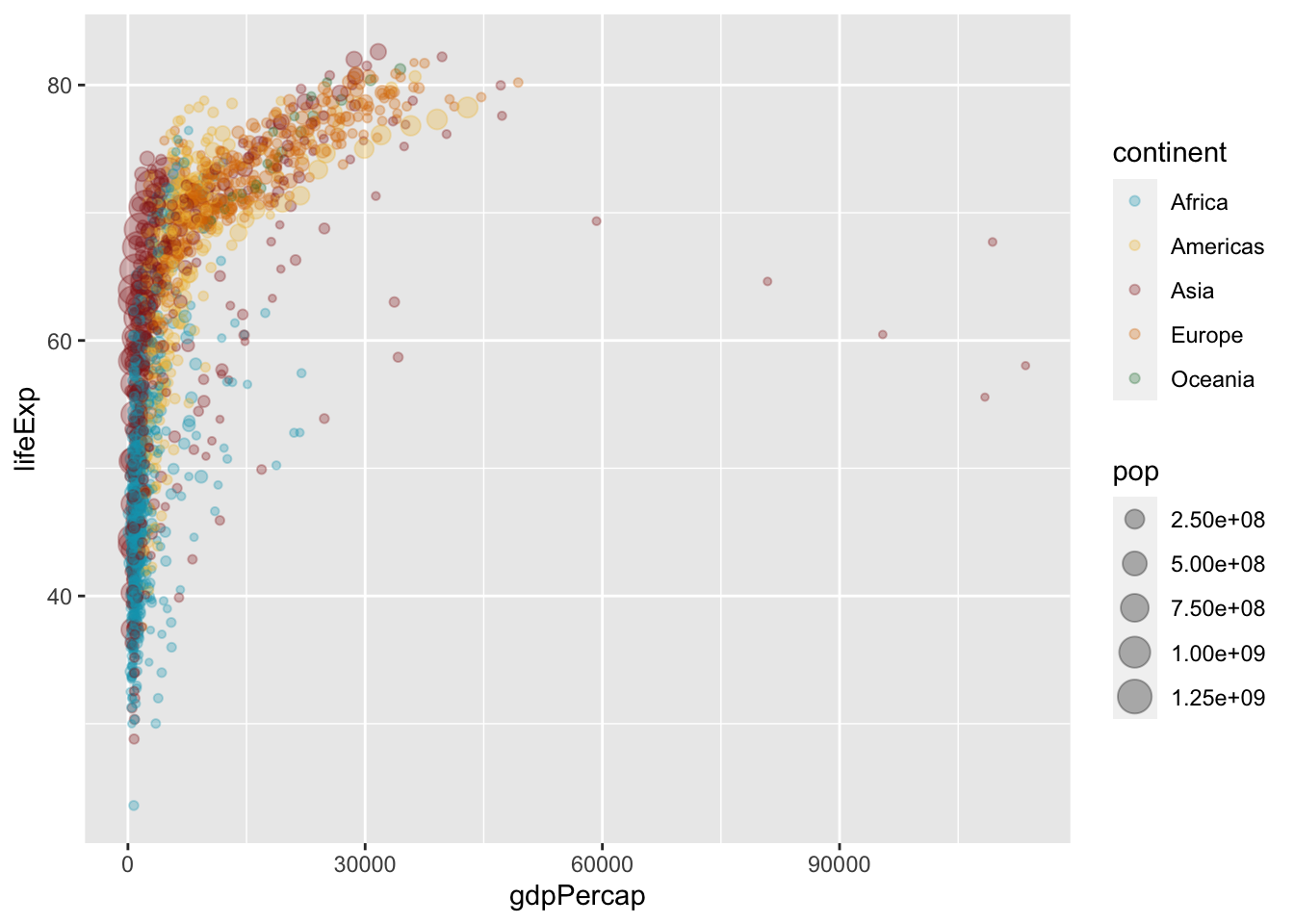## Scales

Let’s load in the package scales.

Code
``library(scales)``

### Filtering

The graph that we have right now actually has duplicate countries! We can fix that by filtering the year to 2007. We’re going to name the different gapminder `gapminder_2007`, like this:

Code
``````gapminder_2007 <- gapminder |>
filter(year == 2007)``````

Now, we need to change the graph so that it is `gapminder_2007` for the data instead of `gapminder`. Now that there are less points, we can have the alpha at full opacity.

Code
``````ggplot(data = gapminder_2007,
mapping = aes(x = gdpPercap,
y = lifeExp,
size = pop,
color = continent)) +
geom_point() +
scale_color_manual(values = met.brewer("Lakota", 5))``````That looks a lot neater and cleaner than it did before! Let’s add some more scales!

## Logarithms

Here, I’m making the x-axis logarithmic to change the axis to be more specific than \$10,000 gaps.

Code
``````ggplot(data = gapminder_2007,
mapping = aes(x = gdpPercap,
y = lifeExp,
size = pop,
color = continent)) +
geom_point() +
scale_color_manual(values = met.brewer("Lakota", 5)) +
scale_x_log10(labels = label_dollar(accuracy = 1),
breaks = c(400, 4000, 40000))``````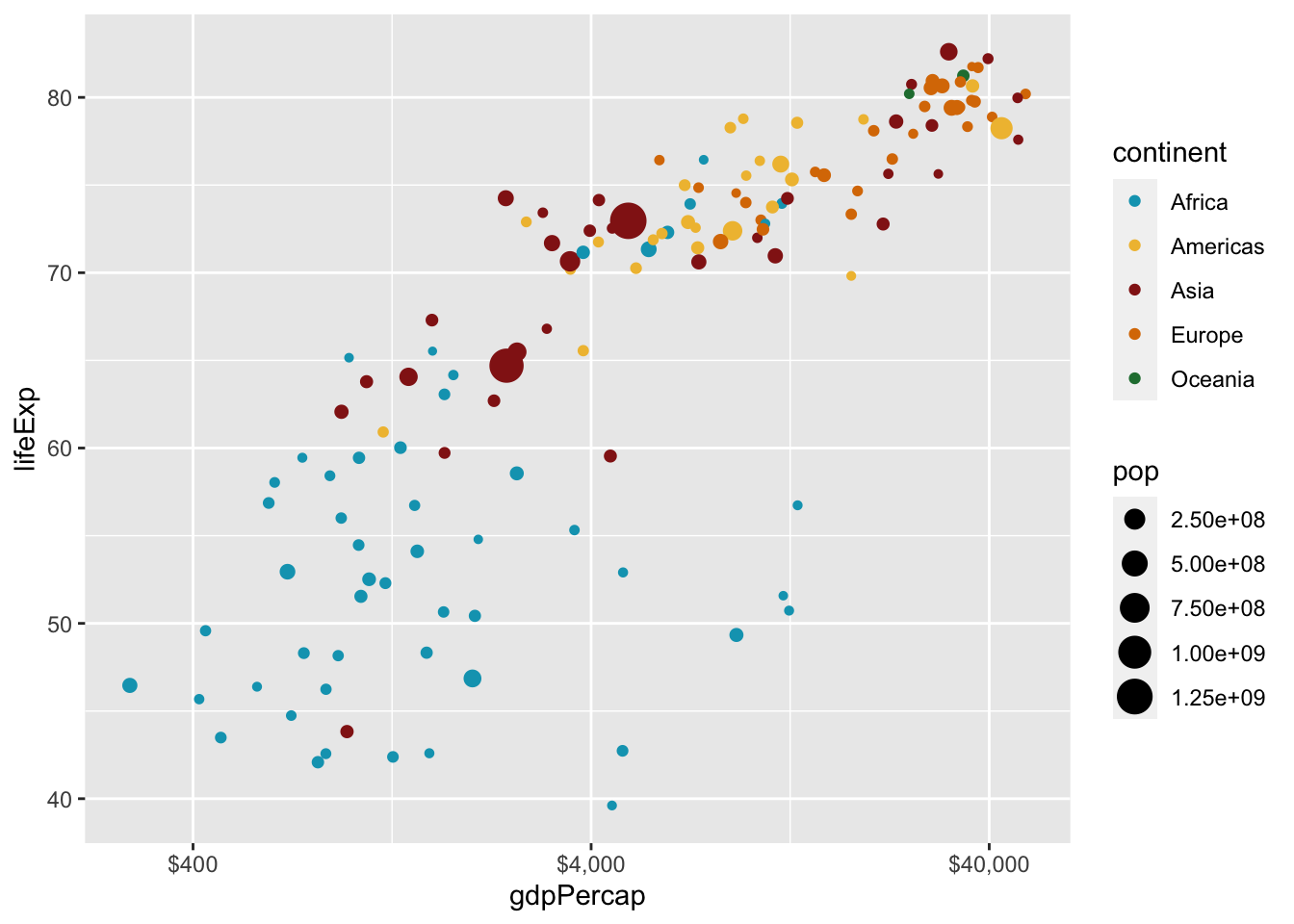## More Scales

Now let’s change the population legend from that ugly NUMBERe+NUMBER, and change it to nicer looking numbers that we can actually read! We do that by adding `scale_size_continuous(labels = comma)` to the code. That will make the size legend have numbers with commas in them.

Code
``````ggplot(data = gapminder_2007,
mapping = aes(x = gdpPercap,
y = lifeExp,
size = pop,
color = continent)) +
geom_point() +
scale_color_manual(values = met.brewer("Lakota", 5)) +
scale_x_log10(labels = label_dollar(accuracy = 1),
breaks = c(400, 4000, 40000)) +
scale_size_continuous(labels = comma)``````## Fixing overplotting without `alpha`

There is still overplotting in this graph! I don’t want to change the `alpha`, because I like the opacity. That is easily fixable with adding just a few things to our code!

### Different shapes in a scatterplot

There are actually 25 different shapes you can use in a scatterplot. We are going to be using shape 21, a circle, with a border. You can access the shapes by typing `pch` into your help page, or `?pch` in your console. The border is important because it can stop confusion about overplotting. The only big change in the code is changing color to fill.

Code
``````ggplot(data = gapminder_2007,
mapping = aes(x = gdpPercap,
y = lifeExp,
size = pop,
fill = continent)) +
geom_point(shape = 21, color = "white") +
scale_fill_manual(values = met.brewer("Lakota", 5)) +
scale_x_log10(labels = label_dollar(accuracy = 1),
breaks = c(400, 4000, 40000)) +
scale_size_continuous(labels = comma)``````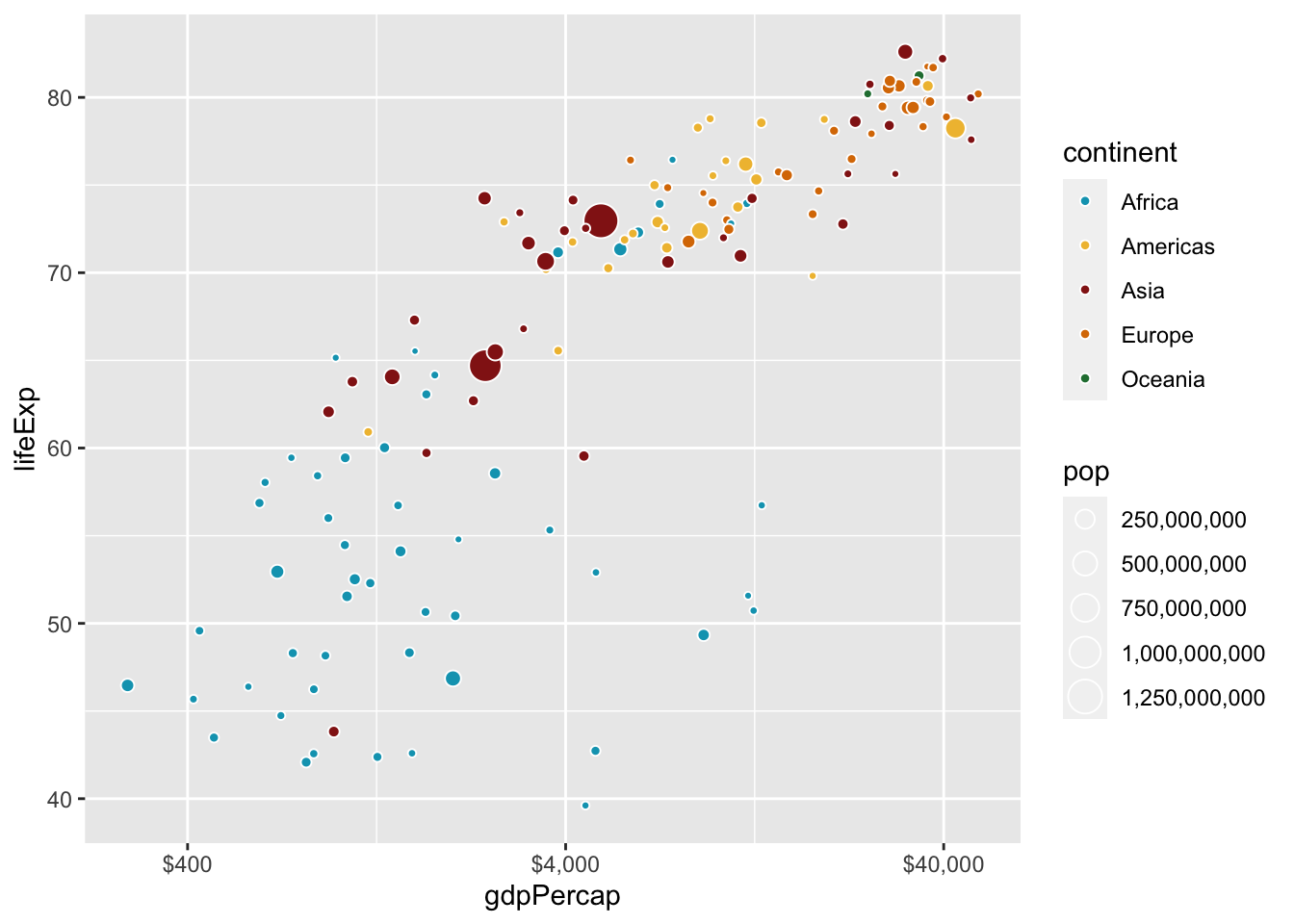I am going to change my theme to `theme_bw`, but I will not cover themes completely in depth. Themes can help change the feel of a graph.

Code
``````ggplot(data = gapminder_2007,
mapping = aes(x = gdpPercap,
y = lifeExp,
size = pop,
fill = continent)) +
geom_point(shape = 21, color = "white") +
scale_fill_manual(values = met.brewer("Lakota", 5)) +
scale_x_log10(labels = label_dollar(accuracy = 1),
breaks = c(400, 4000, 40000)) +
scale_size_continuous(labels = comma)+
theme_bw()``````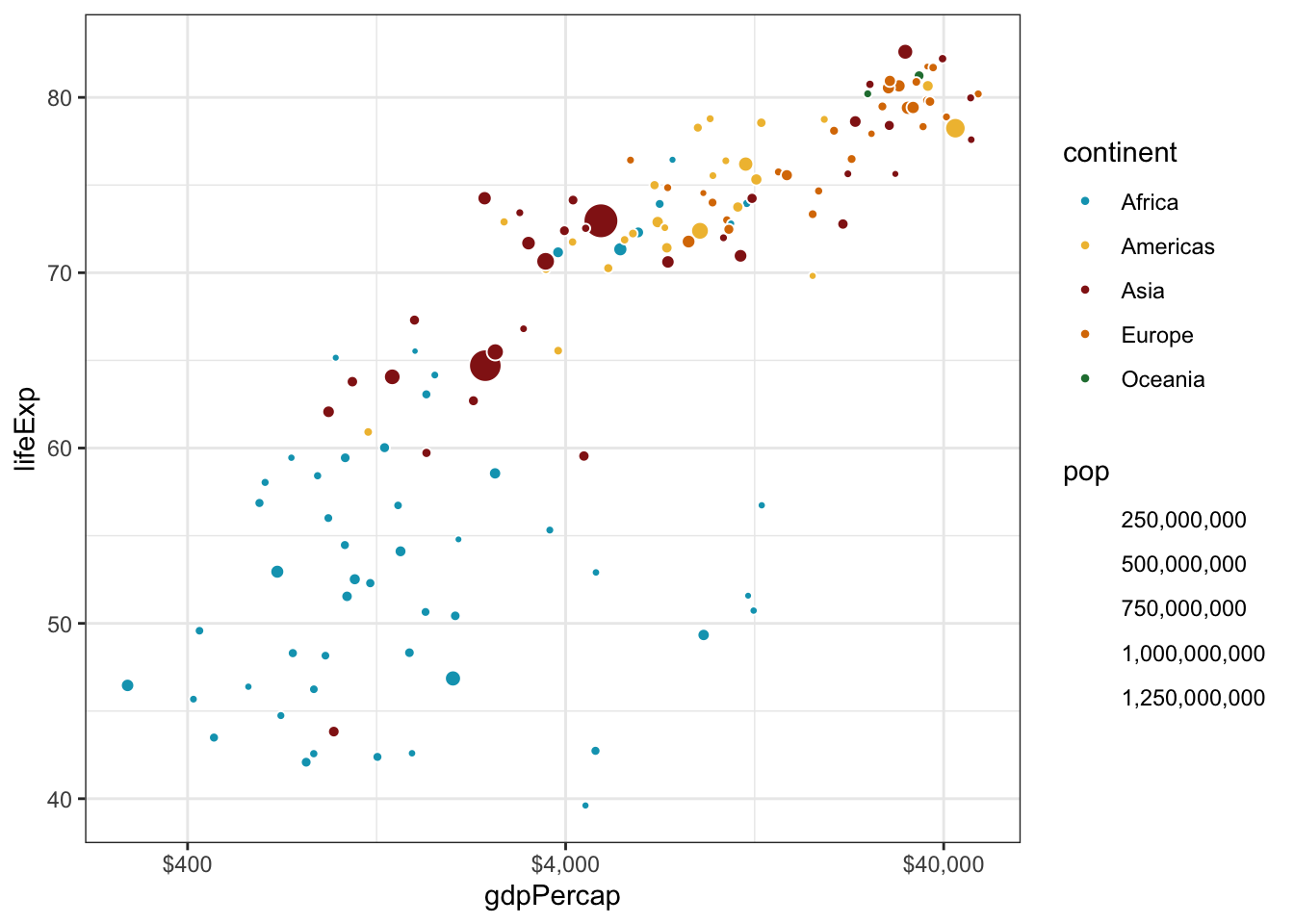## Labels

I am not going to cover the function `labs()` fully, but it is another layer you can add to your plot to add a title, subtitle, caption, legend title, and labels for the x and y axes.

Code
``````ggplot(data = gapminder_2007,
mapping = aes(x = gdpPercap,
y = lifeExp,
size = pop,
fill = continent)) +
geom_point(shape = 21, color = "white") +
scale_fill_manual(values = met.brewer("Lakota", 5)) +
scale_x_log10(labels = label_dollar(accuracy = 1),
breaks = c(400, 4000, 40000)) +
scale_size_continuous(labels = comma) +
theme_bw() +
labs(title = "Gdp and Life Expectancy in 142 countries",
subtitle = "Most of Africa is sick and poor,\nwhile Europe is thriving and rich",
x = "Gdp Per Capita",
y = "Life Expectancy",
fill = "Continent",
size = "Population")``````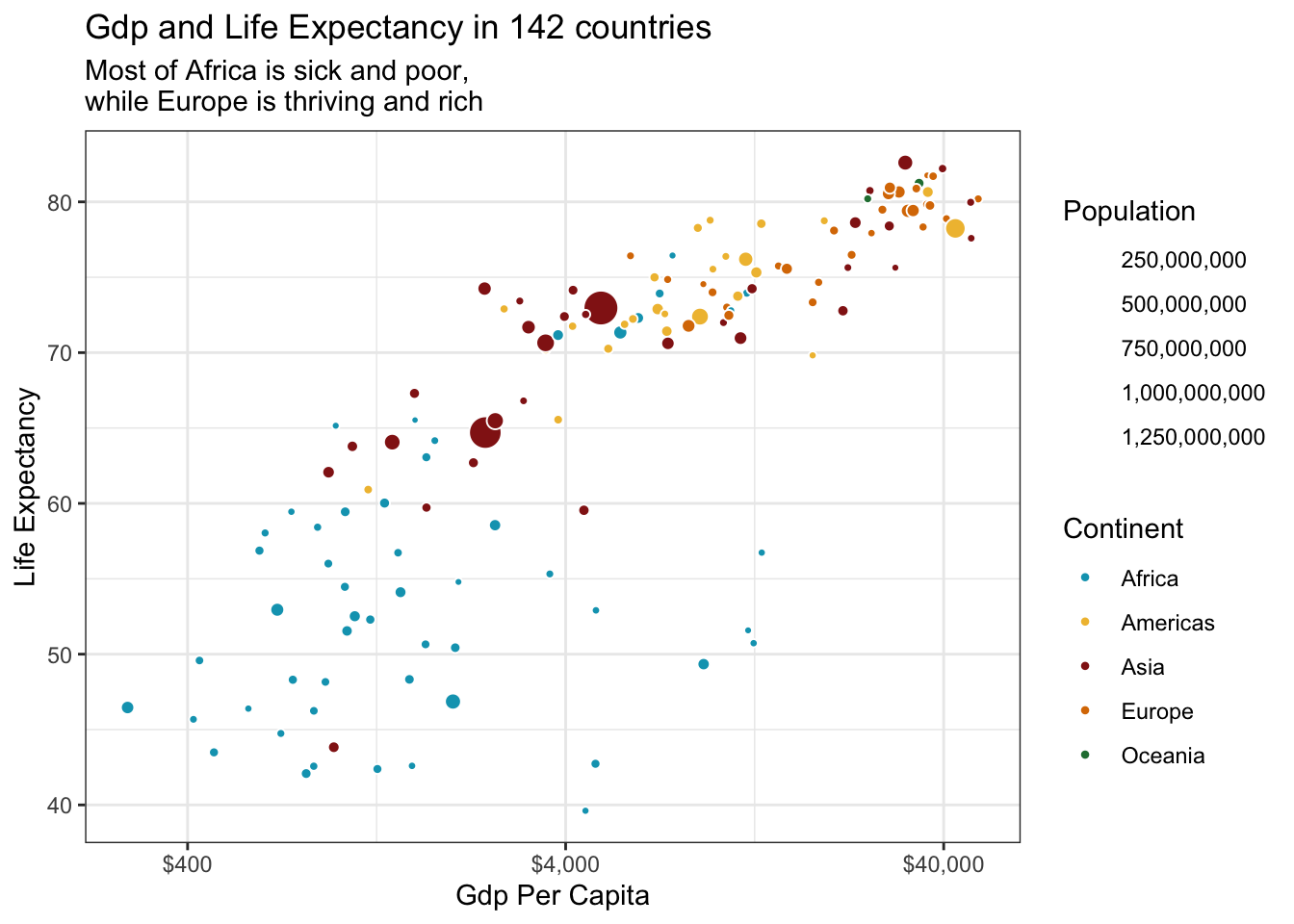## Size of Scales

Wait a minute! Now we can’t see the population guide! We can change that by adding just a couple things to our code. First, we are going to make the legend for the continents just a little bit bigger, so it’s easier to see. We will also make the `scale_size_continuous` color be black.

Code
``````ggplot(data = gapminder_2007,
mapping = aes(x = gdpPercap,
y = lifeExp,
size = pop,
fill = continent)) +
geom_point(shape = 21, color = "white") +
scale_fill_manual(values = met.brewer("Lakota", 5),
guide = guide_legend(override.aes = list(size = 5))) +
scale_x_log10(labels = label_dollar(accuracy = 1),
breaks = c(400, 4000, 40000)) +
scale_size_continuous(labels = comma,
guide = guide_legend(override.aes = list(shape = 19, color = "black"))) +
theme_bw() +
labs(title = "Gdp and Life Expectancy in 142 countries",
subtitle = "Most of Africa is sick and poor,\nwhile Europe is thriving and rich",
x = "Gdp Per Capita",
y = "Life Expectancy",
fill = "Continent",
size = "Population")``````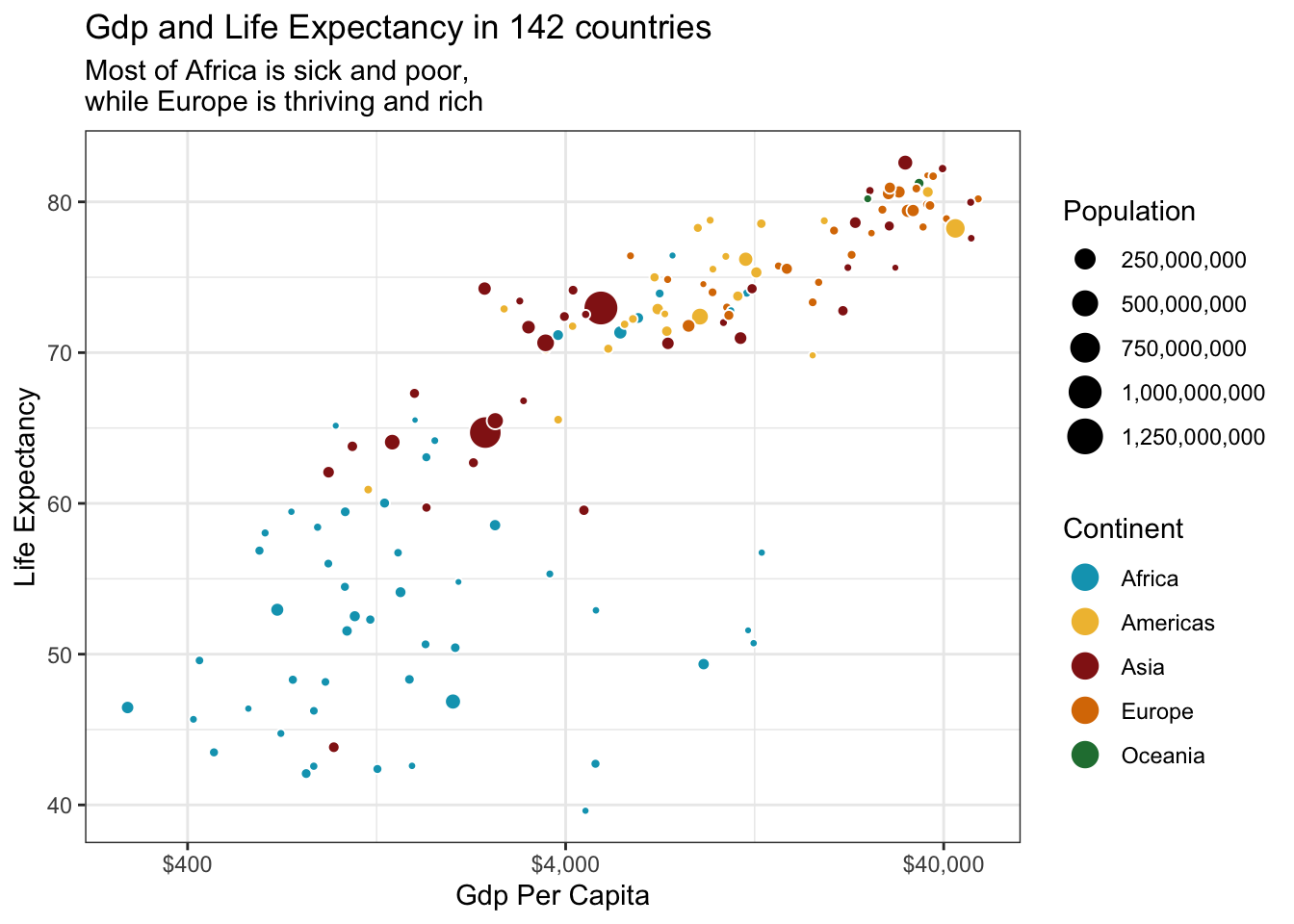## Making it interactive

Now, we need to save the graph to an object. Let’s call that object `cool_plotly_graph`. We also need to add another argument to `aes()`, called `text`. This will make it so we only see the country name, instead of a bunch of random information that we really don’t need.

Code
``````cool_plotly_graph <- ggplot(data = gapminder_2007,
mapping = aes(x = gdpPercap,
y = lifeExp,
size = pop,
fill = continent,
text = country)) +
geom_point(shape = 21, color = "white") +
scale_fill_manual(values = met.brewer("Lakota", 5),
guide = guide_legend(override.aes = list(size = 5))) +
scale_x_log10(labels = label_dollar(accuracy = 1),
breaks = c(400, 4000, 40000)) +
scale_size_continuous(labels = comma,
guide = guide_legend(override.aes = list(shape = 19, color = "black"))) +
theme_bw() +
labs(title = "Gdp and Life Expectancy in 142 countries",
subtitle = "Most of Africa is sick and poor,\nwhile Europe is thriving and rich",
x = "Gdp Per Capita",
y = "Life Expectancy",
fill = "Continent",
size = "Population") ``````

Now that we’ve saved it to an object, we need to add the `ggplotly()` to the code. The tooltip makes it so the `text` argument in `aes` will show up in the `ggplotly()` graph.

Code
``ggplotly(cool_plotly_graph, tooltip = "text")``

`ggplotly` does not support subtitles or multiple legends, so that is why they disappeared.

That’s how you make a basic interactive plot using `ggplotly()`!